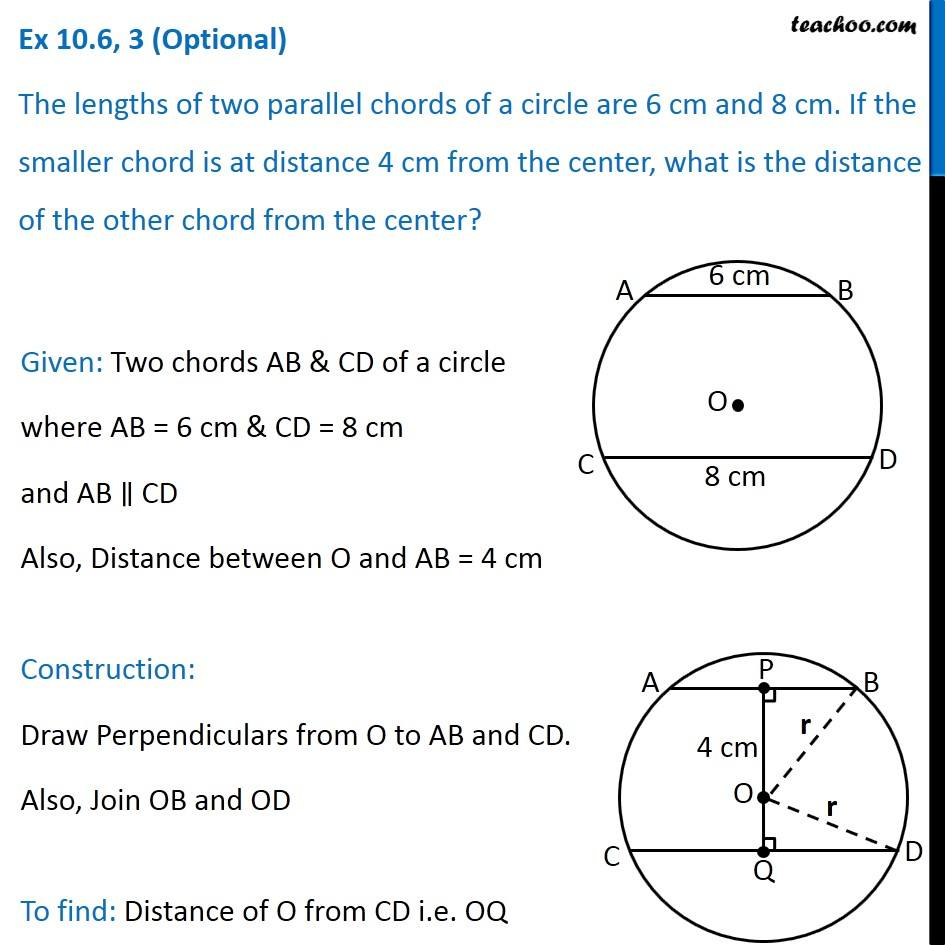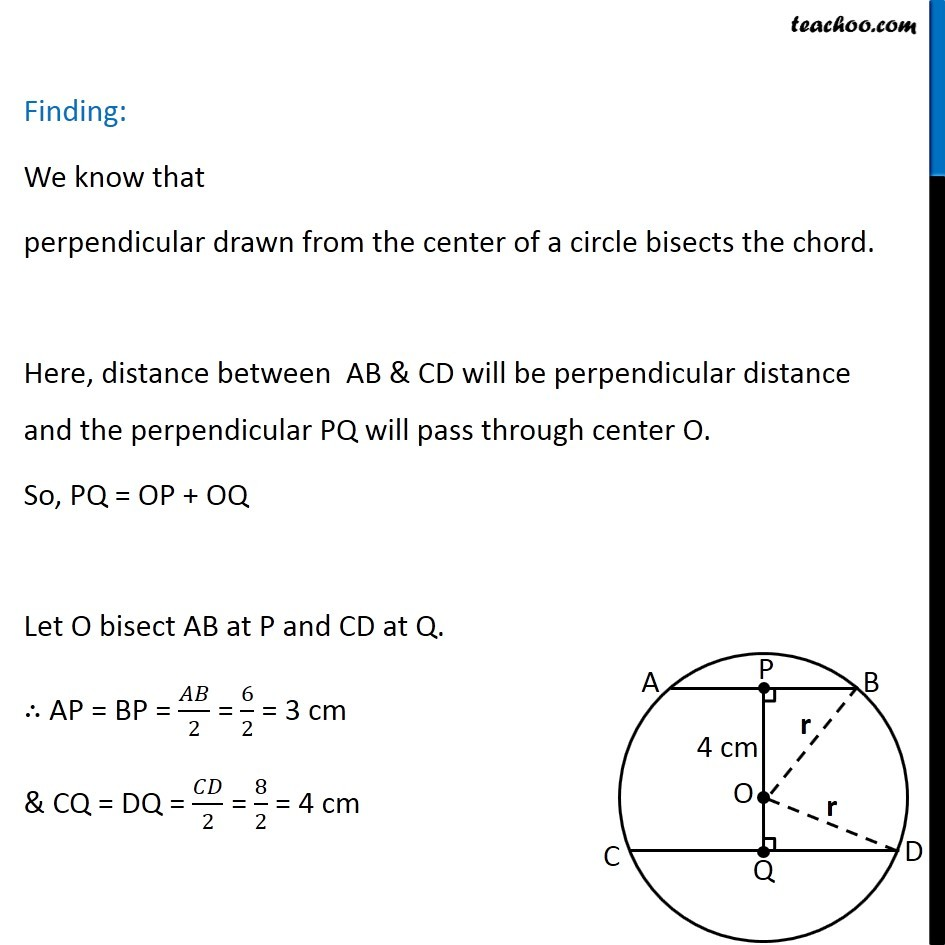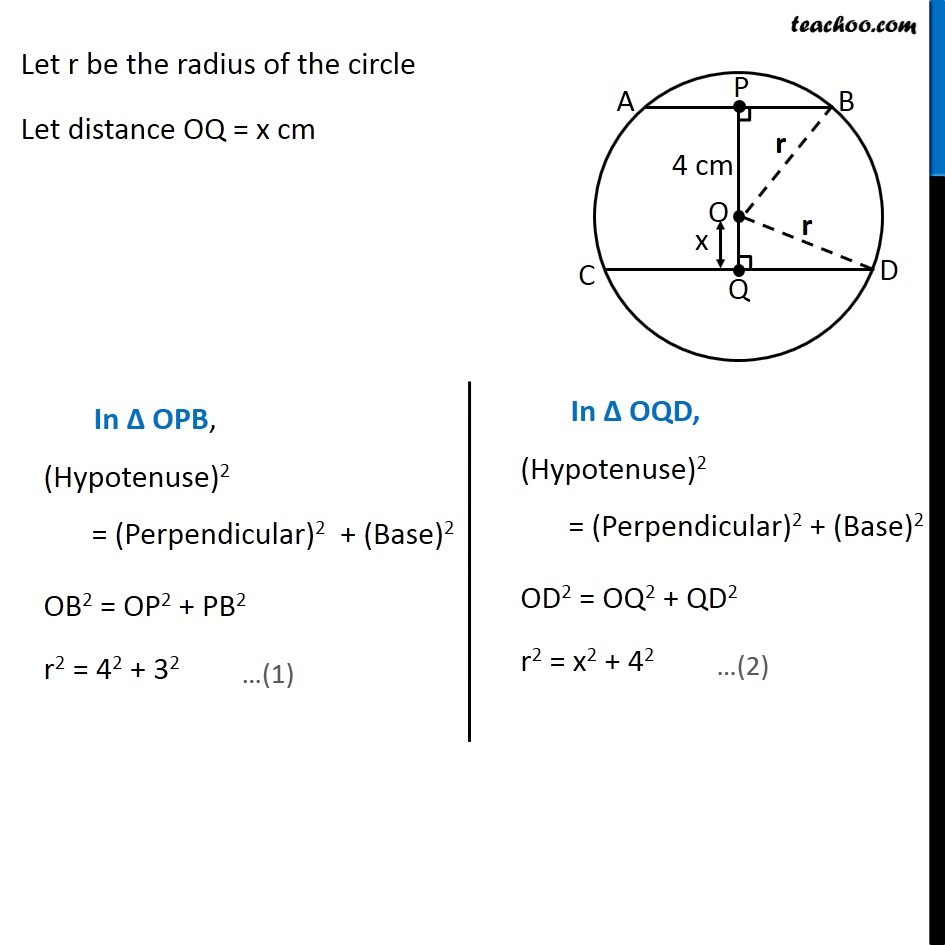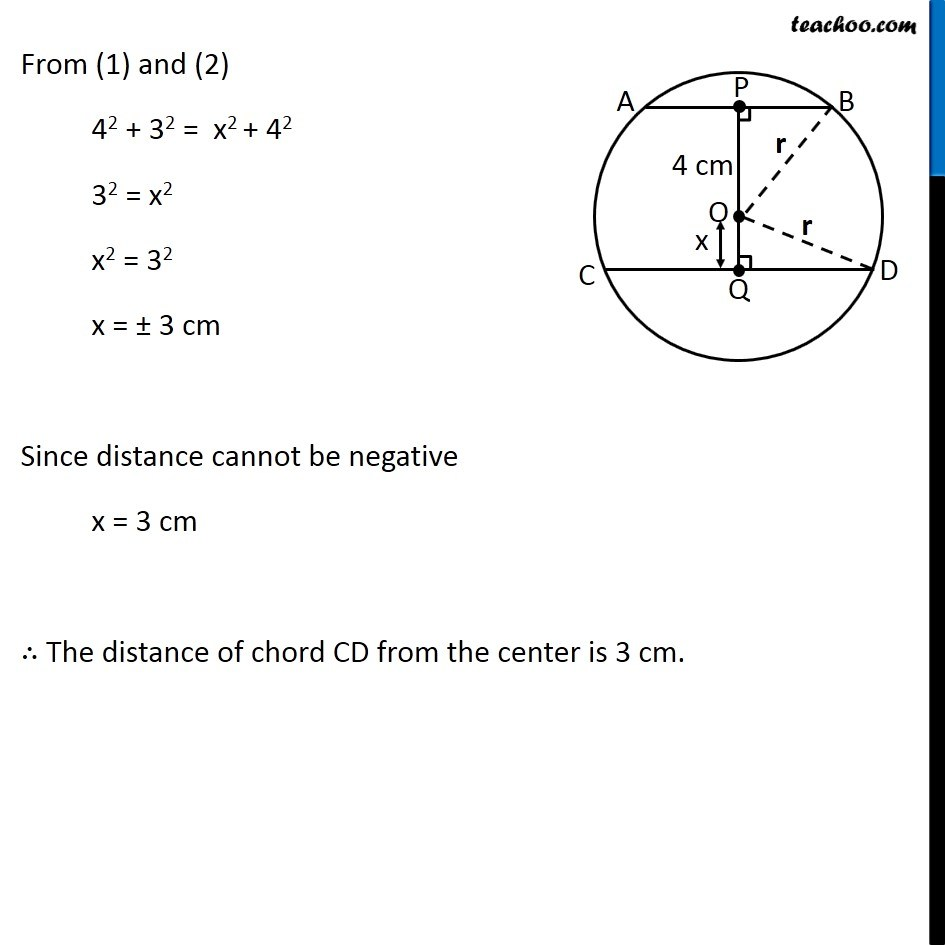Ex 10.6 (Optional)

Chapter 10 Class 9 Circles
Serial order wiseGet live Maths 1-on-1 Classs - Class 6 to 12

### Transcript

Ex 10.6, 3 (Optional) The lengths of two parallel chords of a circle are 6 cm and 8 cm. If the smaller chord is at distance 4 cm from the center, what is the distance of the other chord from the center? Given: Two chords AB & CD of a circle where AB = 6 cm & CD = 8 cm and AB ∥ CD Also, Distance between O and AB = 4 cm Construction: Draw Perpendiculars from O to AB and CD. Also, Join OB and OD To find: Distance of O from CD i.e. OQ Finding: We know that perpendicular drawn from the center of a circle bisects the chord. Here, distance between AB & CD will be perpendicular distance and the perpendicular PQ will pass through center O. So, PQ = OP + OQ Let O bisect AB at P and CD at Q. ∴ AP = BP = 𝐴𝐵/2 = 6/2 = 3 cm & CQ = DQ = 𝐶𝐷/2 = 8/2 = 4 cm Let r be the radius of the circle Let distance OQ = x cm In ∆ OPB, (Hypotenuse)2 = (Perpendicular)2 + (Base)2 OB2 = OP2 + PB2 r2 = 42 + 32 …(1) In ∆ OQD, (Hypotenuse)2 = (Perpendicular)2 + (Base)2 OD2 = OQ2 + QD2 r2 = x2 + 42…(2) From (1) and (2) 42 + 32 = x2 + 42 32 = x2 x2 = 32 x = ± 3 cm Since distance cannot be negative x = 3 cm ∴ The distance of chord CD from the center is 3 cm.